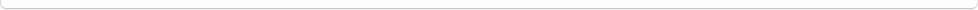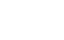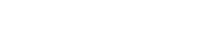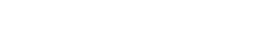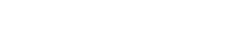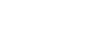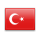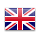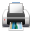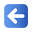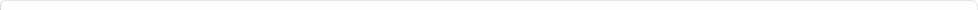Description of Individual Course Units
 Course Unit Code Course Unit Title Type of Course Unit Year of Study Semester Number of ECTS Credits 20150102003101 ENGINEERING MATHEMATICS Compulsory 2 3 5
Level of Course Unit
First Cycle
Objectives of the Course
The aim of this course is to explain some basic concepts of Mathematics and show how to use these concepts in solving certain types of problems which might possibly be encountered in many branches of science and engineering.
Name of Lecturer(s)
Arş. Gör. Dr. Ezgi KAYA
Learning Outcomes
 1 A student defines some mathematical concepts which are essential in his/her field 2 A student defines some mathematical concepts which are essential in his/her field 3 Interprets the relations between mathematical concepts 4 Interprets the relations between mathematical concepts 5 Apply mathematical relationships to solve problems. 6 Apply mathematical relationships to solve problems.
Mode of Delivery
Daytime Class
Prerequisites and co-requisities
Recommended Optional Programme Components
Course Contents
Matrix and determinant operations, Solution of linear equation systems with matrix determinant approaches (Gauss, Gauss-Jordan, Cramer, inverse matrix), vectors, vector operations, scalar and vector products of vectors, orthogonal-orthonormal vectors, linear transformations, eigenvalues and eigenvectors of square matrix, the effect of eigenvalues - eigenvectors on linear system behavior, Algebra of complex numbers, polar representation of complex numbers, derivative of complex functions, analytic functions, Cauchy-Riemann equations,power series, Cauchy integral theorem,Cauchy integral formula, Taylor expansion, Laurent expansion, Residues, residual theorems. Generalized integrals.
Weekly Detailed Course Contents
 Week Theoretical Practice Laboratory 1 Matrix and determinant operations 2 Solution of linear equation systems with matrix determinant approaches(Gauss, Gauss-Jordan, Cramer, inverse matrix) 3 vectors, vector operations, scalar and vector products of vectors 4 orthogonal-orthonormal vectors 5 linear transformations 6 eigenvalues and eigenvectors of square matrix 7 the effect of eigenvalues - eigenvectors on linear system behavior 8 Mid-Term Exam 9 Algebra of complex numbers, polar representation of complex numbers 10 derivative of complex functions 11 analytic functions, Cauchy-Riemann equations,power series 12 Cauchy integral theorem,Cauchy integral formula 13 Taylor expansion, Laurent expansion 14 Residues, residual theorems. Generalized integrals 15 Final Exam
Recommended or Required Reading
1-Dursun Taşçı,Lineer Cebir 2- Aşkın Demirkol, Mühendisler İçin Lineer Sistemler Lineer Cebir - I , Sakarya Kitabevi, 2011. 3- Complex Variables and Their Applications, Addison Wesley, Anthony D. Osborne, 1999. 4- Introduction to Complex Variables and Applications, R.V. Churchill, McGraw-Hill, New York, 1996
Planned Learning Activities and Teaching Methods
Assessment Methods and Criteria
 Term (or Year) Learning Activities Quantity Weight Midterm Examination 1 100 SUM 100 End Of Term (or Year) Learning Activities Quantity Weight Final Examination 1 100 SUM 100 Term (or Year) Learning Activities 40 End Of Term (or Year) Learning Activities 60 SUM 100
Language of Instruction
Work Placement(s)